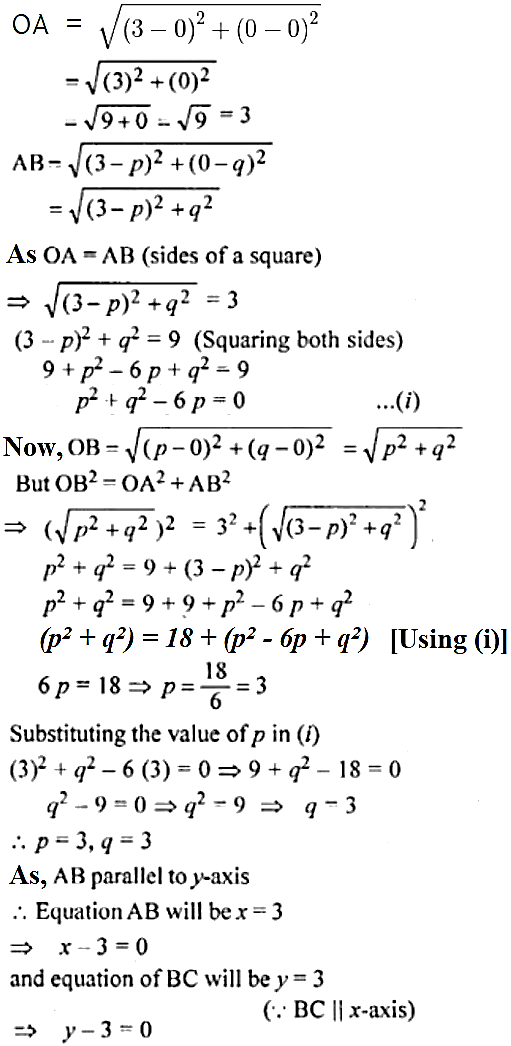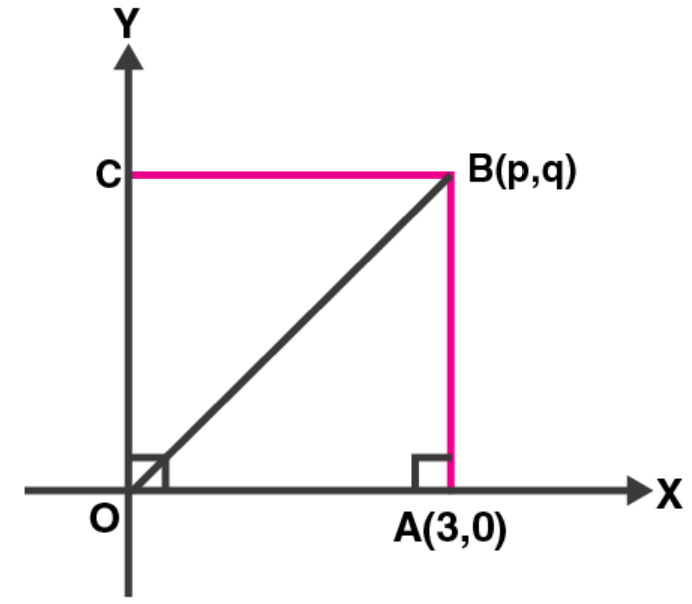Newbie

# OABC is a square, O is the origin and the points A and B are (3, 0) and (p, q). If OABC lies in the first quadrant, find the values of p and q. Also write down the equations of AB and BC.

• 2

An Important Question of M.L Aggarwal book of class 10 Based on Equation of a Straight Line Chapter for ICSE BOARD.
Here a square is given with origin point. and two co-ordinates are also given. Find the solution as per asked in question
This is the Question Number 42, Exercise 12.2 of M.L Aggarwal.

Share

1.This answer was edited.

Given, OABC is a square

Co-ordinates of A and B are (3, 0) and (p, q) respectively

By distance formula, we have• 4